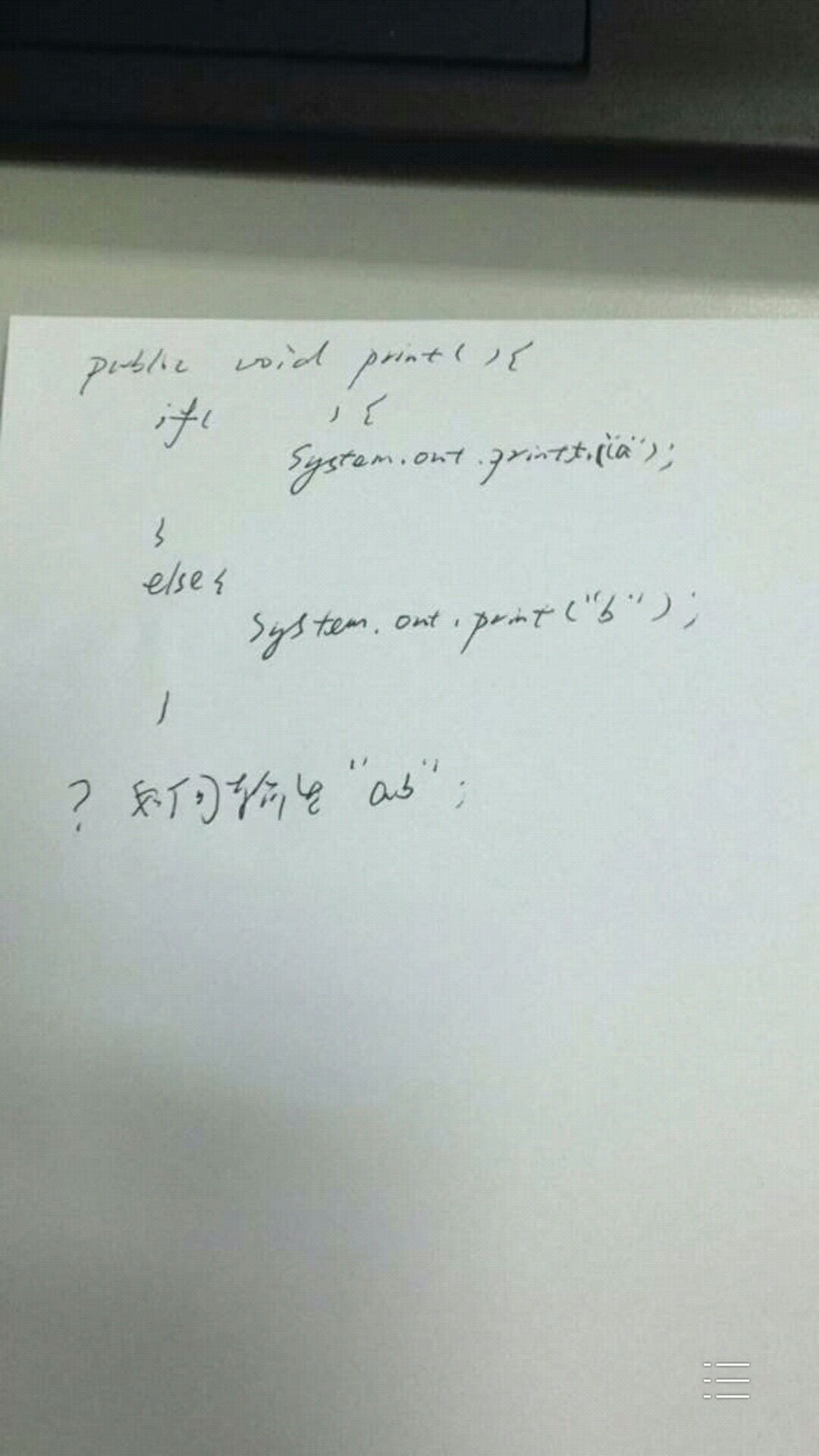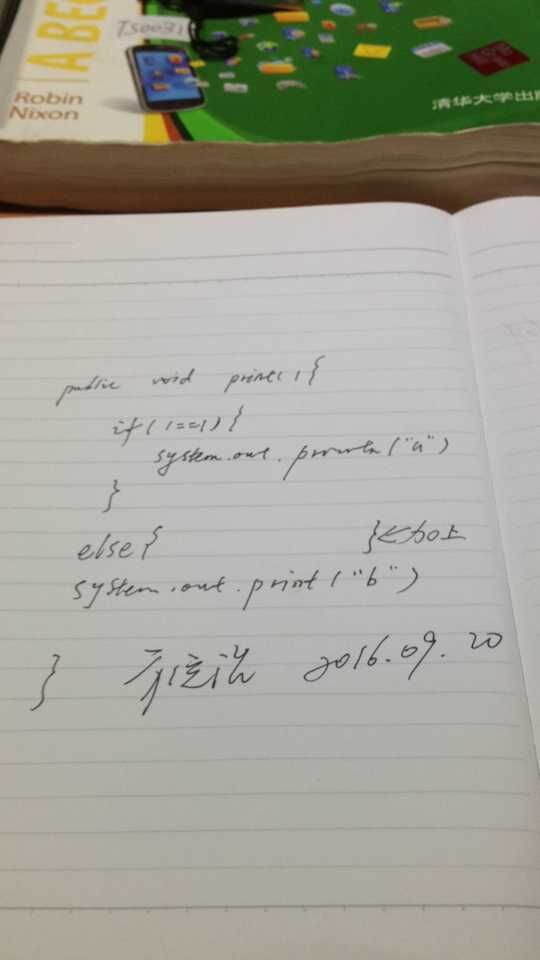# 一道在知乎很火的 Java 题——如何输出 ab

2016/09/20

## 题目## 思路一

### 解法一

``````public void print() {
if (true) {
System.out.print("a");
}

if (false) {
System.out.print("a");
} else {
System.out.print("b");
}
}
``````

## 思路二

### 解法二

print 打印一个值或者对象 void
println 打印并换行 void
printf 格式化打印 PrintStream

``````public void print() {
if (System.out.printf("a") == null) {
System.out.print("a");
} else {
System.out.print("b");
}
}
``````

### 解法三

``````public void print() {
if (new Object() {
boolean print() {
System.out.print("a");
return false;
}
}.print()) {
System.out.print("a");
} else {
System.out.print("b");
}
}
``````

### 解法四

``````public void print() {
if (((BooleanSupplier)(() -> {System.out.print("a");return false;})).getAsBoolean()) {
System.out.print("a");
} else {
System.out.print("b");
}
}
``````

## 脑洞大开

``````public void print() {
//    if ( ) {
System.out.print("a");
//    } else {
System.out.print("b");
//    }
}
``````